• 余弦相似度计算matlab代码计算余弦相似度 张伯超 该脚本将从免疫数据库中读取数据并计算不同特征样本之间的余弦相似度。 用法 -d name of database -s name of subject -f field of the columns used to separate ...
• NULL 博文链接：https://deepfuture.iteye.com/blog/1583934
• 用2.7版本Python实现比较2个文档的相似度计算，采用余弦相似度方法，main函数中的fileName1和fileName2是自己构造的数据集，把这几个文档放在一起就可以运行。
• 文档所给的是余弦相似度计算代码，在文本分类中用来计算相似度值，以此对文本分类
• 余弦相似性：两个向量的夹角越接近于0，其余弦值越接近于1，表面两个向量越相似。**文本相似度计算大致流程：** - 分词 - 合并 - 计算特征值 - 向量化 - 计算向量夹角余弦
1. 使用simhash计算文本相似度 2. 使用余弦相似度计算文本相似度 3. 使用编辑距离计算文本相似度 4. jaccard系数计算文本相似度

2.向量余弦计算文本相似度
2.1 原理
余弦相似性：两个向量的夹角越接近于0，其余弦值越接近于1，表面两个向量越相似。
向量夹角余弦计算：

c

o

s

α

=

x

1

⋅

x

2

+

y

1

⋅

y

2

x

1

2

+

y

1

2

×

x

2

2

+

y

2

2

cos α = \frac{x_1·x_2 + y_1·y_2}{\sqrt{x_1^2+y_1^2}×\sqrt{x_2^2+y_2^2}}

文本相似度计算大致流程：
分词合并计算特征值向量化计算向量夹角余弦值
对于两段文本A和B，对其进行分词，得到两个词列表：

A

=

[

t

1

,

t

2

,

⋯

,

t

i

]

A=[t_1, t_2, \cdots, t_i]

B

=

[

t

1

,

t

2

,

⋯

,

t

j

]

B=[t_1, t_2, \cdots, t_j]

对两个词列表进行合并去重，得到输入样本中的所有词：

T

(

A

,

B

)

=

T

(

A

)

+

T

(

B

)

=

[

t

1

,

t

2

,

⋯

,

t

k

]

T(A,B)=T(A)+T(B)=[t_1,t_2, \cdots , t_k]

计算特征值：
选取词频作为特征值。

F

(

A

)

=

[

f

A

1

,

f

A

2

,

⋯

,

f

A

k

]

F(A)=[f_{A_1},f_{A_2},\cdots,f_{A_k}]

F

(

B

)

=

[

f

B

1

,

f

B

1

,

⋯

,

f

B

k

]

F(B)=[f_{B_1},f_{B_1},\cdots,f_{B_k}]

向量化

A

⃗

=

(

f

A

1

,

f

A

2

,

⋯

,

f

A

k

)

\vec{A}=(f_{A_1},f_{A_2},\cdots,f_{A_k})

B

⃗

=

(

f

B

1

,

f

B

2

,

⋯

,

f

B

k

)

\vec{B}=(f_{B_1},f_{B_2},\cdots,f_{B_k})

计算余弦值：

c

o

s

α

=

∑

i

=

1

k

f

A

i

⋅

f

B

i

∑

i

=

1

k

(

f

A

i

)

2

⋅

∑

i

=

1

k

(

f

B

i

)

2

cos α = \frac{\sum_{i=1}^{k}f_{A_i}·f_{B_i}}{\sqrt{\sum_{i=1}^{k}(f_{A_i})^2}·\sqrt{\sum_{i=1}^{k}(f_{B_i})^2}}

2.2举例
样本1( A )：今天天气真好，适合去逛街，也适合晒太阳。
样本2( B )：今天天气不错，适合去玩，也适合去晒太阳。
样本3( C )：小明不喜欢和小红玩，因为小明不喜欢太阳。
分词：
A=[今天, 天气, 真好, 适合, 去, 逛街, 也, 适合, 晒太阳]
B=[今天, 天气, 不错, 适合, 去, 玩, 也, 适合, 去, 晒太阳]
C=[小明, 不, 喜欢, 和, 小, 红, 玩, 因为, 小明, 不, 喜欢, 太阳]

合并并去重：
[今天, 天气, 真好, 适合, 去, 逛街, 也, 晒太阳, 小明, 不, 喜欢, 和, 小, 红, 玩, 因为, 太阳, 不错]

特征值（词频）计算：
F(A) = [今天:1, 天气:1, 真好:1, 适合:2, 去:1, 逛街:1, 也:1, 晒太阳:1, 小明:0, 不:0, 喜欢:0, 和:0, 小:0, 红:0, 玩:0, 因为:0, 太阳:0, 不错:0]

F(B) = [今天:1, 天气:1, 真好:0, 适合:2, 去:2, 逛街:0, 也:1, 晒太阳:1, 小明:0, 不:0, 喜欢:0, 和:0, 小:0, 红:0, 玩:1, 因为:0, 太阳:0, 不错:1]

F(C) = [今天:1, 天气:1, 真好:0, 适合:2, 去:2, 逛街:0, 也:1, 晒太阳:1, 小明:2, 不:2, 喜欢:2, 和:1, 小:1, 红:1, 玩:2, 因为:1, 太阳:1, 不错:1]

向量化：

A

⃗

=

(

1

,

1

,

1

,

2

,

1

,

1

,

1

,

1

,

0

,

0

,

0

,

0

,

0

,

0

,

0

,

0

,

0

,

0

)

\vec{A}=(1, 1, 1, 2, 1, 1, 1, 1, 0, 0, 0, 0, 0, 0, 0, 0, 0, 0)

B

⃗

=

(

1

,

1

,

0

,

2

,

2

,

0

,

1

,

1

,

0

,

0

,

0

,

0

,

0

,

0

,

1

,

0

,

0

,

1

)

\vec{B}=(1, 1, 0, 2, 2, 0, 1, 1, 0, 0, 0, 0, 0, 0, 1, 0, 0, 1)

C

⃗

=

(

1

,

1

,

0

,

2

,

2

,

0

,

1

,

1

,

2

,

2

,

2

,

1

,

1

,

1

,

2

,

1

,

1

,

1

)

\vec{C}=(1, 1, 0, 2, 2, 0, 1, 1, 2, 2, 2, 1, 1, 1, 2, 1, 1, 1)

计算余弦：

c

o

s

A

⃗

B

⃗

=

0.8058229640253802

cos \vec{A}\vec{B}=0.8058229640253802

c

o

s

A

⃗

C

⃗

=

0.0

cos \vec{A}\vec{C}=0.0

c

o

s

B

⃗

C

⃗

=

0.06299407883487121

cos \vec{B}\vec{C}=0.06299407883487121

A

⃗

B

⃗

\vec{A}\vec{B}

的夹角的余弦更趋近于1，所以相似度更高。
2.3 总结
余弦相似度对于短文本的相似度计算还是比较准确的，但是对于大文本计算时，速度不如simhash快。
以下测试分别通过simhash和余弦相似度计算相似度的时间：
字符数simhash耗时/ms余弦相似度耗时/ms201.70.42004.21.8200020.010.72000024.134.0200000176.7668.5
另外测试了10000个字符，步长100的线性数据，绘制结果如下：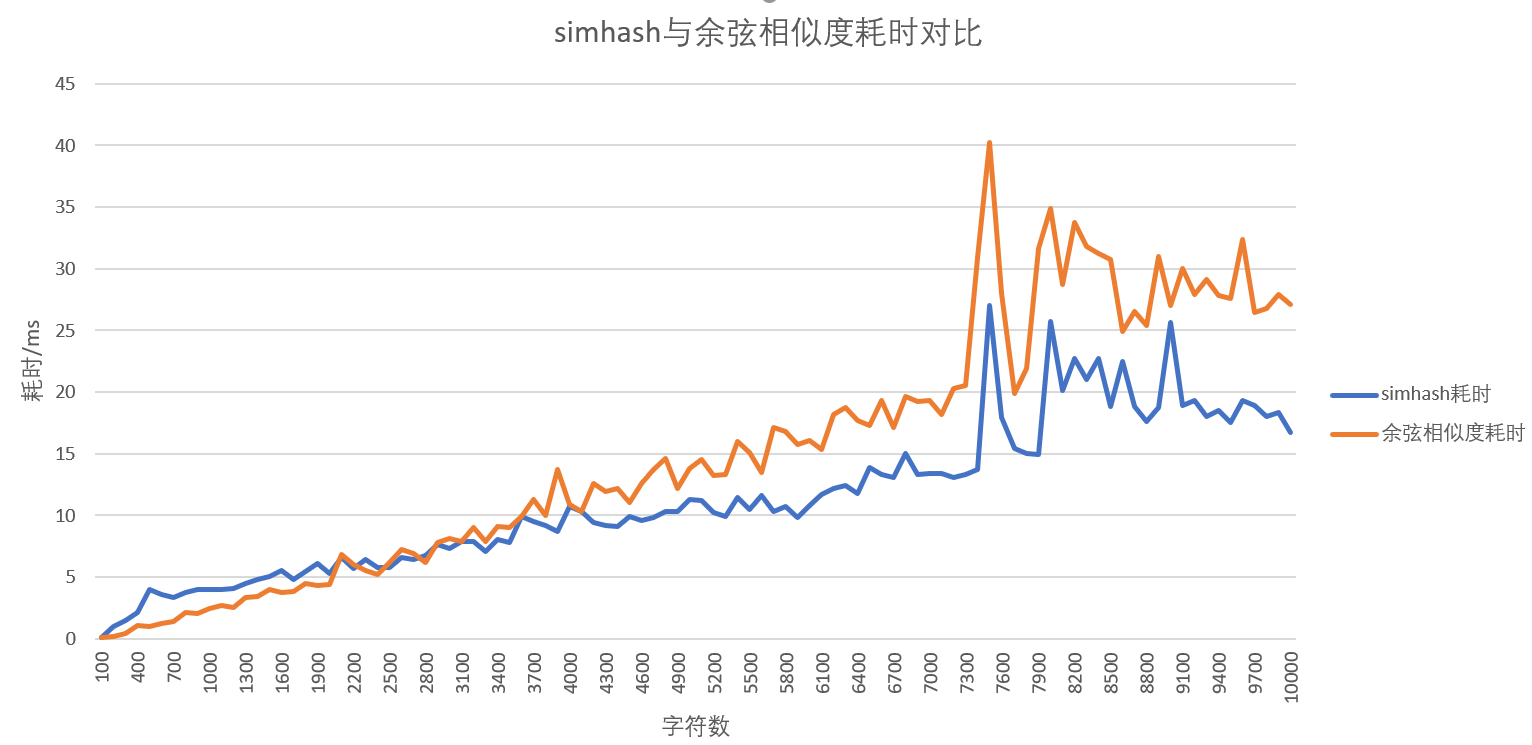当字符数量大约大于3000时，simhash的效率高于余弦相似度的相率。（中间有段时间突增是因为启动了其他程序，占用了CPU导致的）
所以短文本使用余弦相似度来计算文本相似度还是比较适合的。而对于准确度来说，这两种方法的准确度差不多，最主要的还是取决于特征值或者的计算方式。通过简单的词频计算作为特征值，虽然简单，但是仅仅只能通过词语本身来衡量其特性，而没有语境（即上下文）来更准确的确定一个词的特征。因此也演变出了一些新的优化方法或者模型，例如TD-IDF等等，后面再陆续总结下。

All efforts, only for myself, no longer for others 
展开全文• 有多个文本，分别是正负样本，使用余弦相似度计算负样本与正样本的样本相似度，若准确率高，后期可判断新加样本与正样本的相似度。 输入如下所示（存入txt）： content label 今天下午，在龙口市诸由观镇涧村张常鸿...
项目需求
有多个文本，分别是正负样本，使用余弦相似度计算负样本与正样本的样本相似度，若准确率高，后期可判断新加样本与正样本的相似度。
输入如下所示： content label 今天下午，在龙口市诸由观镇涧村张常鸿家的大院里。 1 呼啦呼啦，巴拉巴拉小魔仙 1 张常鸿的爸爸张振俭告诉记者，从4月份以后就再没有见到张常鸿了。 0 张常鸿2000年2月14日出生于山东烟台龙口市。 0 大家好 0 在上午举行的资格赛中，选手将以跪射、卧射和立射三个姿势各打40发。 0 呼啦呼啦，巴拉巴拉小魔仙 0 你好 0
切词
分贝使用jieba.lcut和tfidf进行分词，代码如下所示：
# -*- coding:utf-8 -*-
# @time  : 11:20
# @Author:xxma
import jieba
import jieba.analyse as ana
import re
def cn(text):
'''
剔除特殊字符及数字，只保留中文
:param text:
:return:
'''
str = re.findall(u"[\u4e00-\u9fa5]+", text)
return str

# 加载停用词
with open(r'D:\xxma\文本分析\停用词.txt', 'r', encoding='utf-8') as f:
stopwords = [s.strip() for s in f.readlines()]

def jiebacut(text):
"""
使用普通分词
:param text: 原始文本
:return:切分后的词，且用空格分隔
"""
text=str(cn(text))
words = [w for w in jieba.lcut(text) if w not in stopwords]  # 剔除停用词
# print(words,len(words))
words = [w for w in words if len(words) > 2] #剔除短文本
return ' '.join(words)

def tfidfcut(text):
"""
使用tfidf分词
:param text:原始文本
:return:切分后的词，且用空格分隔
"""
text = str(cn(str(text)))
ana.set_stop_words(r'D:\xxma\文本分析\停用词.txt')
# print(ana.extract_tags(text))
words = [w for w in ana.extract_tags(text) if len(ana.extract_tags(text)) > 1]
return ' '.join(words)


生成词向量，并计算余弦相似度
分别使用词频和tfidf生成词向量，再分别计算余弦相似度，代码如下：
# -*- coding:utf-8 -*-
# @time  : 11:21
# @Author:xxma
"""
输入必须有2列，分别为content 及label
label为1或0：其中1为正样本，即问题样本，0为负样本，即待验证的样本，
"""
import pandas as pd
import numpy as np
from sklearn.feature_extraction.text import CountVectorizer, TfidfTransformer
from sklearn.feature_extraction.text import TfidfVectorizer
from sklearn.metrics.pairwise import cosine_similarity

"""
1、CountVectorizer：会将文本中的词语转换为词频矩阵，它通过fit_transform函数计算各个词语出现的次数,通过get_feature_names()可获得所有文本
的关键词，通过toarray()可看到词频矩阵的结果，TfidfTransformer用于统计vectorizer中每个词语的TFIDF值
2、TfidfVectorizer：将原始文档的集合转化为tf-idf特性的矩阵，相当于CountVectorizer配合TfidfTransformer使用的效果。
即TfidfVectorizer类将CountVectorizer和TfidfTransformer类封装在一起
"""
from 文本分析.分词 import jiebacut, tfidfcut

# 加载数据
# df = df.sort_values(by='label',ascending=False)
threshold = float(input('请输入余弦相似度的阈值(0-1之间的相似度):'))
print('加载数据ing')
df2 = pd.read_csv(r'D:\xxma\文本分析\sd\data-1626936455161.csv', sep=',').drop_duplicates() # 问题样本数据
df2 = df2.loc[0:50000, ('content', 'label')]
df = pd.concat([df1, df2])
df = df.sort_values(by='label', ascending=False)
# 分词
print('开始分词ing')
df['cut'] = df['content'].apply(tfidfcut)
df['len'] = df['cut'].apply(len)
df = df[df['len'] > 0].reset_index(drop=True)
# 正负样本个数
positive_num = df[df['label'] == 1].shape
negitive_num = df[df['label'] == 0].shape
# 使用词频生成词向量
print('生成词向量ing')
Vectorizer = CountVectorizer()
vector = Vectorizer.fit_transform(df['cut'])
names = Vectorizer.get_feature_names()
positive = vector.toarray()[0:positive_num]
negitive = vector.toarray()[positive_num:]

# 使用tfidf生成词向量
Vectorizer_tfidf = TfidfVectorizer()
vector_tfidf = Vectorizer_tfidf.fit_transform(df['cut'])
names_tfidf = Vectorizer_tfidf.get_feature_names()
positive_tfidf = vector_tfidf.toarray()[0:positive_num]
negitive_tfidf = vector_tfidf.toarray()[positive_num:]

# 余弦相似度
print('计算余弦相似度ing')
consine_fre = cosine_similarity(negitive, positive)
consine_tfidf = cosine_similarity(negitive_tfidf, positive_tfidf)

# 数据保存
print('保存结果数据ing')
cos = [consine_fre, consine_tfidf]
cos_name = ['consine_fre', 'consine_tfidf']
writer = pd.ExcelWriter(r'D:\xxma\文本分析\sd\consine_result.xlsx')
for name, i in zip(cos_name, cos):
df_result = pd.DataFrame(i)
df_result['max'] = df_result.max(axis=1)
df_result['max_index'] = np.argmax(i, axis=1)

question = df[(df['label'] == 1)]['content'].reset_index(drop=True)
df_result['matched'] = df_result['max_index'].apply(lambda x: question[x])
# 合并原始数据
orgin_df = pd.DataFrame(df[(df['label'] == 0)]['content'].reset_index(drop=True))
consine_df = df_result.loc[:, ('max', 'matched')]
result_df = orgin_df.join(consine_df)
result_df = result_df.sort_values(by='max', ascending=False)
result_df = result_df[result_df['max'] > threshold]
result_df.to_excel(writer, sheet_name=name, index=0, encoding='utf-8')
writer.save()
#writer.close()
#最终发现：使用tfidf进行分词，再使用词频生成词向量的效果最佳

展开全文自然语言处理
• 1、余弦函数 余弦函数在三角形中的计算公式 在直角坐标系中 向量a和向量b之间的距离我们用向量c表示，就是上图中的黄色直线，那么向量c在直角坐标系中的长度...余弦相似度算法：一个向量空间中两个向量夹角间的余弦
搬运自https://zhuanlan.zhihu.com/p/43396514
1、余弦函数
余弦函数在三角形中的计算公式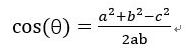在直角坐标系中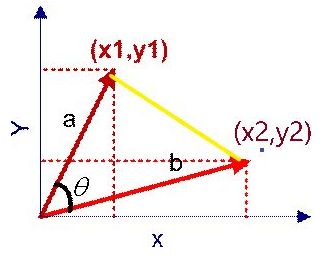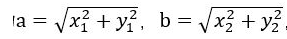向量a和向量b之间的距离我们用向量c表示，就是上图中的黄色直线，那么向量c在直角坐标系中的长度为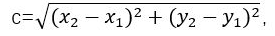将a，b，c带入三角函数的公式中得到如下的公式：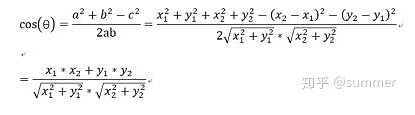这是2维空间中余弦函数的公式，那么多维空间余弦函数的公式就是：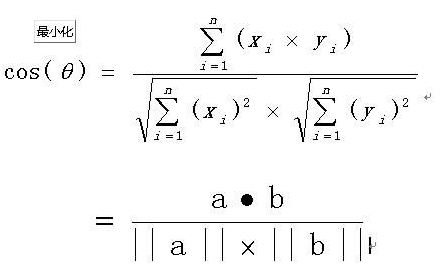2、余弦相似度
余弦相似度量：计算个体间的相似度。 相似度越小，距离越大。相似度越大，距离越小。
余弦相似度算法：一个向量空间中两个向量夹角间的余弦值作为衡量两个个体之间差异的大小，余弦值接近1，夹角趋于0，表明两个向量越相似，余弦值接近于0，夹角趋于90度，表明两个向量越不相似。
下面我们介绍使用余弦相似度计算两段文本的相似度。思路：1、分词；2、列出所有词；3、分词编码；4、词频向量化；5、套用余弦函数计量两个句子的相似度。
句子A：这只皮靴号码大了。那只号码合适。
句子B：这只皮靴号码不小，那只更合适。
1、分词：使用结巴分词对上面两个句子分词后，分别得到两个列表：
listA=[‘这‘, ‘只‘, ‘皮靴‘, ‘号码‘, ‘大‘, ‘了‘, ‘那‘, ‘只‘, ‘号码‘, ‘合适‘]
listB=[‘这‘, ‘只‘, ‘皮靴‘, ‘号码‘, ‘不小‘, ‘那‘, ‘只‘, ‘更合‘, ‘合适‘]
2、列出所有词。将listA和listB放在一个set中，得到：
set={‘不小’, ‘了’, ‘合适’, ‘那’, ‘只’, ‘皮靴’, ‘更合’, ‘号码’, ‘这’, ‘大’}
将上述set转换为dict，key为set中的词，value为set中词出现的位置，即‘这’:1这样的形式。
dict1={‘不小’: 0, ‘了’: 1, ‘合适’: 2, ‘那’: 3, ‘只’: 4, ‘皮靴’: 5, ‘更合’: 6, ‘号码’: 7, ‘这’: 8, ‘大’: 9}，可以看出“不小”这个词在set中排第1，下标为0。
3、将listA和listB进行编码。将每个字转换为出现在set中的位置，转换后为：
listAcode=[8, 4, 5, 7, 9, 1, 3, 4, 7, 2]
listBcode=[8, 4, 5, 7, 0, 3, 4, 6, 2]
我们来分析listAcode，结合dict1，可以看到8对应的字是“这”，4对应的字是“只”，9对应的字是“大”，就是句子A和句子B转换为用数字来表示。
4、对listAcode和listBcode进行oneHot编码。就是计算每个分词出现的次数。oneHot编号后得到的结果如下：
listAcodeOneHot = [0, 1, 1, 1, 2, 1, 0, 2, 1, 1]
listBcodeOneHot = [1, 0, 1, 1, 2, 1, 1, 1, 1, 0]
下图总结了句子从分词，列出所有词，对分词进行编码，计算词频的过程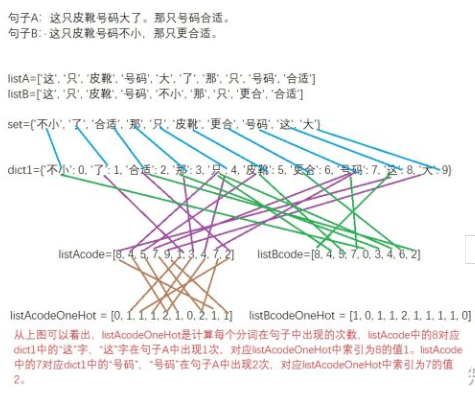5、得出两个句子的词频向量之后，就变成了计算两个向量之间夹角的余弦值，值越大相似度越高。
listAcodeOneHot = [0, 1, 1, 1, 2, 1, 0, 2, 1, 1] listBcodeOneHot = [1, 0, 1, 1, 2, 1, 1, 1, 1, 0]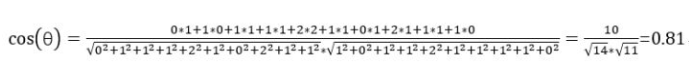代码如下：
// An highlighted block
import jieba
import math
s1 = '这只皮靴号码大了。那只号码合适'
s1_cut = [i for i in jieba.cut(s1, cut_all=True) if i != '']
s2 = '这只皮靴号码不小，那只更合适'
s2_cut = [i for i in jieba.cut(s2, cut_all=True) if i != '']
print(s1_cut)
print(s2_cut)
word_set = set(s1_cut).union(set(s2_cut))
print(word_set)

word_dict = dict()
i = 0
for word in word_set:
word_dict[word] = i
i += 1
print(word_dict)

s1_cut_code = [word_dict[word] for word in s1_cut]
print(s1_cut_code)
s1_cut_code = *len(word_dict)

for word in s1_cut:
s1_cut_code[word_dict[word]]+=1
print(s1_cut_code)

s2_cut_code = [word_dict[word] for word in s2_cut]
print(s2_cut_code)
s2_cut_code = *len(word_dict)
for word in s2_cut:
s2_cut_code[word_dict[word]]+=1
print(s2_cut_code)

# 计算余弦相似度
sum = 0
sq1 = 0
sq2 = 0
for i in range(len(s1_cut_code)):
sum += s1_cut_code[i] * s2_cut_code[i]
sq1 += pow(s1_cut_code[i], 2)
sq2 += pow(s2_cut_code[i], 2)

try:
result = round(float(sum) / (math.sqrt(sq1) * math.sqrt(sq2)), 2)
except ZeroDivisionError:
result = 0.0
print(result)

展开全文• 今天小编就为大家分享一篇Python 余弦相似度与皮尔逊相关系数 计算实例，具有很好的参考价值，希望对大家有所帮助。一起跟随小编过来看看吧
• faiss常用的两个相似度搜索是L2欧氏距离搜索和余弦距离搜索（注意不是余弦相似度） 简单的使用流程： import faiss index = faiss.IndexFlatL2(d) # 建立L2索引,d是向量维度 index = faiss.IndexFlatIP(d) # 建立In
faiss
faiss常用的两个相似度搜索是L2欧氏距离搜索和余弦距离搜索（注意不是余弦相似度）
简单的使用流程：
import faiss
index = faiss.IndexFlatL2(d)  # 建立L2索引,d是向量维度
index = faiss.IndexFlatIP(d) # 建立Inner product索引
D,I = index.search(test, k) # (D.shape = test.shape * k, I同理)

上述代码实现了对于test向量（也可以是矩阵）索引train中L2距离最近的k个向量，返回其具体distance和索引index
IndexFlatIP（）函数实现的是余弦距离的计算也就是

x

y

t

xy^t

,显然，当向量范数不为一的情况下不能等同于余弦相似度

x

y

t

∣

∣

x

∣

∣

∣

∣

y

∣

∣

\frac{xy^t}{||x||||y||}

在许多论文特别是需要计算索引的时候，相似度往往选择余弦相似度，因此在这里记录一下如何实现：
cosine similarity 实现
train = np.array([[1.0,1.0],[2.5,0],[0,2.5],[1.5,0.5]]).astype('float32') # 注意 必须为float32类型
test = np.array([[0.5,0.5]]).astype('float32')
print('L2 norm of train', np.linalg.norm(train))
print('L2 norm of test', np.linalg.norm(test))
faiss.normalize_L2(train)
faiss.normalize_L2(test)
print('L2 norm of train', np.linalg.norm(train))
print('L2 norm of test', np.linalg.norm(test))

L2 norm of train 1.4142135
L2 norm of test 0.70710677
L2 norm of train 0.99999994
L2 norm of test 0.99999994

对于被索引矩阵和查询向量，都先经过L2归一化，(normlize_L2函数)
定义索引函数
def KNN_cos(train_set, test_set, n_neighbours):
index = faiss.IndexFlatIP(train_set.shape)
D, I = index.search(test_set, n_neighbours)
return D,I


测试
Distance, Index = KNN_cos(train, test,3)

Distance: (array([[0.99999994, 0.8944272 , 0.70710677]], dtype=float32),
Index: array([[0, 3, 2]]))

在github上看到有人给出这样的解决方法
num_vectors = 1000000
vector_dim = 1024
vectors = np.random.rand(num_vectors, vector_dim)

#sample index code
quantizer = faiss.IndexFlatIP(1024)
index = faiss.IndexIVFFlat(quantizer, vector_dim, int(np.sqrt(num_vectors)), faiss.METRIC_INNER_PRODUCT) # 利用IVFFLat提升效率
train_vectors = vectors[:int(num_vectors/2)].copy()
faiss.normalize_L2(train_vectors)
index.train(train_vectors)
faiss.normalize_L2(vectors)
#index creation done

#let's search
query_vector = np.random.rand(10, 1024)
faiss.normalize_L2(query_vector)
D, I = index.search(query_vector, 100)

print(D)

其实这里做了个提速：利用IVFlat先进行聚类再索引，提升效率，详见可以看官方源码
总结
关于faiss库进行索引查询还有很多操作，特别是对于海量数据，合理的利用faiss可以极大提升效率。`
展开全文python 机器学习 开发语言
• , [1.4013e-45, 0.0000e+00]]]]) 1: 什么是余弦相似度 余弦相似度可以理解为：a 投影在b上，重合越多那么相似度越大。在三角函数中，要想|a| * |b| * con ab之间的夹角 的积越大，则需要夹角余弦趋近于1，也就是角...
• 目录 一、余弦相似度计算方式 1、python 2、sklearn 3、scipy 4、numpy 5、pytorch 6、faiss 二、规模暴增计算加速 1、numpy矩阵计算GPU加速——cupy 2、pytorch框架cuda加速 3、faiss的加速方法 总结 在做文本匹配...算法 人工智能 python
• 首先，大致梳理以下余弦相似度方法和IF-IDF方法的原理 1、余弦相似度计算文档相似度 余弦相似度方法的原理公式其实与我们高中的时候所学到的余弦公式相似，
• 本文中根据甲方需求实现调用GPU加速计算两千万次的余弦相似度计算，根据需求分析，可以大致分为两个实现目标。 在10s内完成1：2000000次的余弦相似度计算。 在10s内同时完成topk的计算。 二、实现方法 因为在需求...
• pdist(S1，‘cosine’)计算S1中所有行组合之间的余弦距离。因此，所有组合之间的相似性为1 - pdist(S1，‘cosine’)。 我们可以将其转换为一个方阵，其中元素(i,j)对应于第i行和第j行之间的相似性，使用squareform(1...数据挖掘 机器学习
• 使用scipy库： from scipy.spatial.distance import pdist def cosine_sim_1(x, y): X=np.vstack([x,y]) d1=1-pdist(X,'cosine') return d1 使用numpy方法： ...import numpy as np def cosine_sim_2(x, y): ...python
• 那么在图数据库Neo4j中如何进行余弦相似度计算呢？下面我会简单说明整个计算过程。不得不说我们非常幸运，Neo4j的插件提供了这样的一个功能，让我们能够简单、直接的在其上实现相似度计算。 首先我们来进行环境...知识图谱
•算法
• 余弦相似度计算字符串相似率 功能需求：最近在做通过爬虫技术去爬取各大相关网站的新闻，储存到公司数据中。这里面就有一个技术点，就是如何保证你已爬取的新闻，再有相似的新闻 或者一样的新闻，那就不存储到...
• 余弦相似度用向量空间中两个向量夹角的余弦值作为衡量两个个体间差异的大小。 余弦值越接近1，就表明夹角越接近0度，也就是两个向量越相似，这就叫"余弦相似性"。 我们知道，对于两个向量，如果他们之间的夹角越...
• 余弦相似度计算，使用scipy.spatial.distance引入模块pdlist函数使用 引入模块 from scipy.spatial.distance import pdist pdlist函数使用 a=[1,1,1] b=[1,1,2] c=[1,1,2] d=[1,1,2] e=[1,1,2] # cos print("cos:",...python
• 智能信息检索——利用SIMNOMERGE余弦相似度计算文档得分的算法实现1．实验目的2．实验任务与要求3．实验说明书⑴功能描述⑵概要设计⑶详细设计⑷代码实现4．实验成果 《信息检索导论》部分实验python实现汇总请进入...算法 python 人工智能 信息检索
• reference：... # -*- coding:utf-8 -*- # 余弦计算相似度度量 http://blog.csdn.net/u012160689/article/details/15341303 import math import re import datetim...
• Matlab余弦相似度算法判断图片相似度并识别源代码 Matlab 余弦相似度 图像匹配 可直接运行
• 余弦相似度计算： \cos(\bf{v_1}, \bf{v_2}) = \frac{\left( v_1 \times v_2 \right)}{||v_1|| * ||v_2|| } \cos(\bf{M_1}, \bf{M_2}) = \frac{\left(M_1 \times M_2^T \right)}{||M_1|| \times ||M_1||^T } ### ...
• 一. 向量基本知识点 1 向量乘积公式 2 向量模计算公式 ...二. 余弦相似度计算数学原理 ...在工作中一直使用余弦相似度算法计算两段文本的相似度和...本文还通过一个例子演示如果使用余弦相似度计算两段文本的相似度...
• Java 实现推荐系统 两个字符串 余弦相似度 算法。...